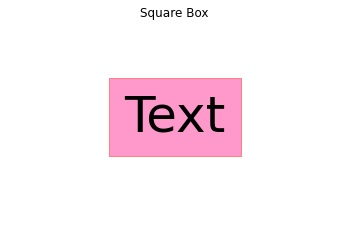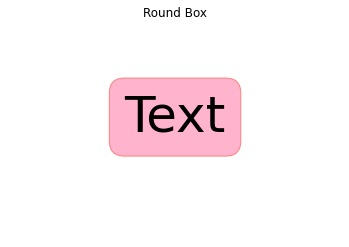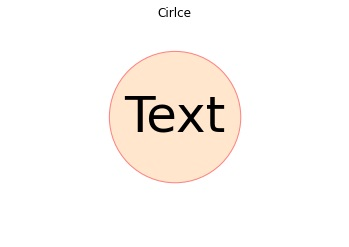# Python | Text Box in Plane Figure

In this tutorial, we are going to learn how to draw a text box in a plane figure i.e. without any axis?
Submitted by Anuj Singh, on August 16, 2020

It is rarely used but again, in some cases where we need only to show a text box, we can do so by using the function matplotlib.pyplot.axis(Flase).

Illustrations:## Python code for text box in plane figure

```import matplotlib.pyplot as plt

# Square
plt.figure()
plt.text(0.35,0.5, "Text", size=50,
bbox=dict(boxstyle="square",
ec=(1., 0.5, 0.5),
fc=(1., 0.6, 0.8),
)
)
plt.title('Square Box')
plt.axis(False)
plt.show()

# Rounded
plt.figure()
plt.text(0.35, 0.5, "Text", size=50,
bbox=dict(boxstyle="round",
ec=(1., 0.5, 0.5),
fc=(1., 0.7, 0.8),
)
)
plt.title('Round Box')
plt.axis(False)
plt.show()

# Circle
plt.figure()
plt.text(0.35, 0.5, "Text", size=50,
bbox=dict(boxstyle="circle",
ec=(1., 0.5, 0.5),
fc=(1., 0.9, 0.8),
)
)
plt.title('Cirlce')
plt.axis(False)
plt.show()
```

Output:

```Output is as Figure
```